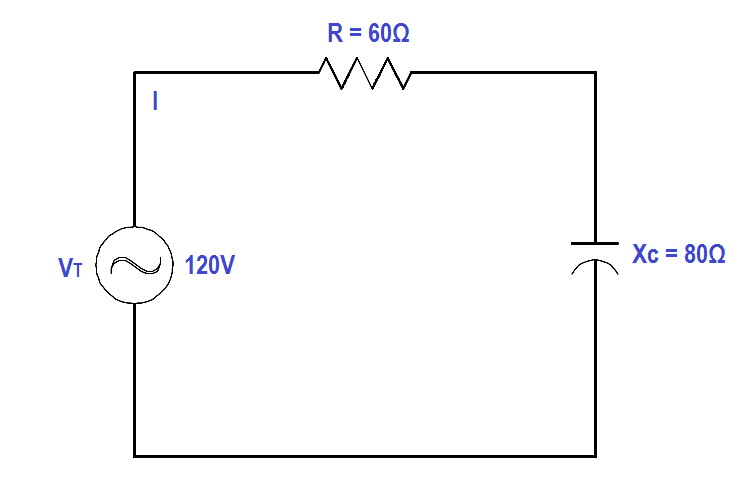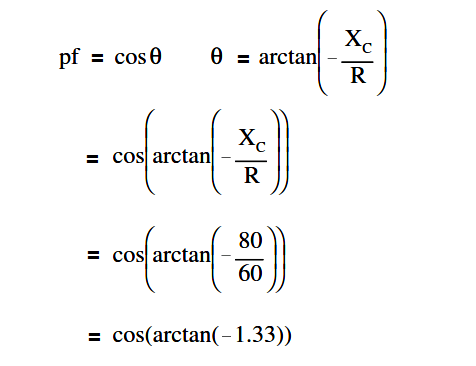# Calculate Power in Series RC Circuit

An 80 Ω XC and a 60 Ω resistance are in series with a 120V source, as shown in Figure.Figure : Series R-C Circuit

Find:

1. Z
2. Current, IT
3. Power Factor, pf
4. True Power, P
5. Reactive Power, Q
6. Apparent Power, S

Solution :

Z = √R2 + XC2

Z = √602 + 802

Z = 100 Ω

I= VT/Z

I= 120/100

I= 1.2 amps

##### 2. Calculate Power factor (pf)p.f. = cos (- 53º)

p.f. = 0.6

##### 3. Calculate True Power, P

P = EI cos θ

P = (120)(1.2)(0.6)

P = 86.4 watts

##### 4. Calculate Reactive Power, Q

Q = EI sin θ

Q = (120)(1.2)(0.798)

Q = 114.9 VAR

S = EI

S = (120)(1.2)

S = 144 VA